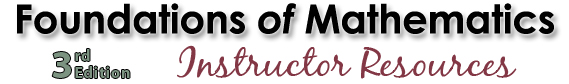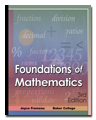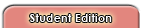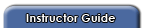All files are in Adobe .pdf format. Get Adobe Reader

Section

 Chapter 1 — Whole Numbers Section 1.1 Introduction to Whole Numbers Section 1.2 Rounding Whole Numbers Section 1.3 Adding and Subtracting Whole Numbers Section 1.4 Multiplying Whole Numbers Section 1.5 Dividing Whole Numbers Applications Practice Test Chapter 2 — Decimal Numbers Section 2.1 Reading, Writing, Comparing, and Rounding Decimal Numbers Section 2.2 Adding and Subtracting Decimal Numbers Section 2.3 Multiplying Decimal Numbers Section 2.4 Dividing Decimal Numbers Applications Practice Test Chapter 3 — Fractions Section 3.1 Introduction to Fractions Section 3.2 Finding Prime Factors Section 3.3 Reducing Fractions Section 3.4 Multiplying and Dividing Fractions and Mixed Numbers Section 3.5 Building Equivalent Fractions and Ordering Fractions Section 3.6 Adding and Subtracting Fractions and Mixed Numbers Applications Practice Test Chapter 4 — Ratios and Proportions Section 4.1 Ratios Section 4.2 Proportions Section 4.3 Applications of Proportions Applications Practice Test Chapter 5 — Percents Section 5.1 Converting between Percents, Decimals, and Fractions Section 5.2 Solving Percent Problems Applications Practice Test Chapter 6 — Signed Numbers, Exponents, and Order of Operations Section 6.1 Adding, Subtracting, Multiplying, and Dividing Signed Numbers Section 6.2 Exponents Section 6.3 Evaluating Expressions Using the Order of Operations Applications Practice Test Answer Keys Demonstrate Your Understanding Methodologies (Example 2 Problems) Chapter Applications Additional Exercises Practice Tests:     Chapter 1   Chapter 2   Chapter 3   Chapter 4   Chapter 5   Chapter 6
 © 2008 Pacific Crest